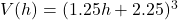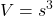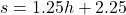## A cube has an edge of 2.25 feet. The edge is increasing at the rate of 1.25 feet per hour. Express the volume of the cube as a function of h

Question

A cube has an edge of 2.25 feet. The edge is increasing at the rate of 1.25 feet per hour. Express the volume of the cube as a function of h, the number of hours elapsed.

in progress 0
6 months 2021-07-19T09:17:34+00:00 1 Answers 43 views 0Step-by-step explanation:

Recall that the volume of a cube is given by:Where s is the side length of the cube.

The edges of the cube has an original length of 2.25 feet. It increases by 1.25 feet per hour. In other words, the length s after h hours can be modeled by the equation:Substitute. Hence, our function is: